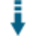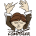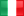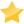# Log Delta Time: Log the time difference between PHP statementsDownload .zip
 InfoExampleView files (9)Download .zip Reputation Support forum (1) Blog Links
 Ratings Unique User Downloads Download Rankings Not enough user ratings Total: 163 All time: 8,891 This week: 106Version License PHP version Categories log-delta-time 1.0.0 GNU General Publi... 5.4 PHP 5, Logging, Performance and optim...
Description

Author

This class can log the time difference between PHP statements.

It can write or append to a log file messages passed at different points of execution of a PHP script.

The class can output to the log file the message and the difference of time between the current and the previous log call.

Optionally it can log messages only if the time that elapsed since the last call exceeds a given minimum period.

The class can also log values of variables to identify better related log calls.

Recommendations

Create a logger to an application
I need a logger class to insert in file or database.

Innovation AwardJanuary 2016 Number 5Prize: One downloadable copy of CodeLobster Professional Logging information is useful to understand what happened and when in an application. However, when you are profiling your application, trying to determine the sections of your code that are taking too long, you do not need the information about code that executed in an acceptable length of time. This class can help finding code that is taking too long just by logging messages of statements that exceeded a given minimum execution time. Manuel LemosPerformance Level
Name: Classes: Alessandro Quintiliani `` 3 packages by Alessandro QuintilianiItaly 54 2557 98 in Italy58626 in ItalyLevel 1Innovation awardNominee: 2x

## Example

 ``` .  *  * ***************************************************************************************************  */ \$thisPHPscriptName = basename(\$_SERVER['PHP_SELF']); // this instruction simply generates mainexample.php \$scriptPHPtoDebug = ""; include("Class.LogDeltaTime.php"); #### CLASS TO DEBUG THIS PHP SCRIPT ### if (\$_POST['btn_mainexample'] == \$thisPHPscriptName) {             \$scriptPHPtoDebug = \$thisPHPscriptName;         define(MYVAR_VALUE, 10); // set a constant MYVAR_VALUE only as an example     define(MAX_FACTORIAL, 14); // set a constant MAX_FACTORIAL only as an example     define(DELTA_MIN_TO_TRACK, 2); // set minimum time difference in seconds (constant named i.e. DELTA_MIN_TO_TRACK) between two consecutive log messages to be tracked to the log file     define(MIN_EXECUTION_DELAY, 2); // set min delay execution script, in seconds (constant named i.e.MIN_EXECUTION_DELAY)     define(MAX_EXECUTION_DELAY, 4); // set max delay execution script, in seconds (constant named i.e.MAX_EXECUTION_DELAY)         \$LOGDIR = "log"; # directory containing the log generated by this PHP script when instantiating LogDeltaTime class. It's created only if not exists at the same level of this PHP script     \$LOGFILE = "logmainexample.txt"; # log file created, if not exists, inside \$LOGDIR         # instance of LogDeltaTime class. This instance also sets the start microtime execution of this PHP script     \$log = new LogDeltaTime(\$LOGDIR, \$LOGFILE, 1); // replace the value of the third parameter with 2 to append the statements to logmainexample.txt after each run of mainexample.php         \$log->wlog ( "first statement" );         \$log->wlog ( "initializing myvar and c variables" );     \$myvar = rand ( 7, MYVAR_VALUE );     \$c = 0;         \$log->wlog ( "check if myvar=" . MYVAR_VALUE . ": if so, a block code with a sum instruction is executed" );     \$log->wlog ( "begin 'if' block having myvar as a control variable and executed only if myvar=" . MYVAR_VALUE );         \$log->setDeltaLog(false); // from this point on, the log statement format doesn't have delta time         \$log->wlog ( "value of myvar: \$myvar" );     if (\$myvar == MYVAR_VALUE) {         \$log->wlog ( "entered the block code having myvar=\$myvar" );                 \$log->wlog ( "first log message in the 'if' block having myvar=\$myvar" );         \$log->wlog ( "set values of a and b" );         \$a = rand ( -5, 9 );         \$b = rand ( -5, 9 );                 \$log->wlog ( "executing simple sum between a=\$a and b=\$b and the result placed in c" );         \$c = \$a + \$b;         \$log->wlog ( " c (= a + b) equals \$c" );                 \$log->wlog ( "last log message in the 'if' block having myvar=\$myvar as a control variable" );     }     \$log->wlog ( "end 'if' block having myvar as a control variable and executed only if myvar=" . MYVAR_VALUE );         \$log->setDeltaLog(true); // from this point on, the log statement format is switched back to that having delta time         \$log->wlog ( "begin new if block having c as a control variable used as input to calculate its factorial number (allowed values of c: [0-" . MAX_FACTORIAL . "])" );     \$log->wlog ( "value of c: \$c" );     if (\$c < 0) {         \$log->wlog ( "first log message in the 'if' block having c as a control variable" );         \$log->wlog ( " c=\$c: cannot calculate the factorial of a negative number" );         \$factorial = "undefined";     } elseif (\$c > MAX_FACTORIAL) {         \$log->wlog ( "first log message in the if block having c>" . MAX_FACTORIAL );         \$log->wlog ( " c=\$c: " . MAX_FACTORIAL . " is the maximum allowed value for c" );     } else {           \$log->wlog ( "first log message in the if block having c>=0 and c<=" . MAX_FACTORIAL );             \$log->wlog ( " c=\$c (c > 1 and c <= " . MAX_FACTORIAL . "): its factorial value can be calculated" );             if (\$c <= 1) {               \$log->wlog ( "c=\$c --> c!=1 " );               \$factorial = 1;            } else {                 \$factorial = \$c;                 \$log->wlog ( "begin for loop to calculate \$c! in an iterative way" );                 for(\$i = \$c; \$i > 2; \$i --) {                     \$stepfact = \$c - \$i + 1;                     \$log->wlog ( "execution factorial step \$stepfact" );                     \$factorial *= (\$i - 1);                     \$log->wlog ( "intermediate factorial value: \$factorial" );                 }                 \$log->wlog ( "end for loop with factorial=\$factorial" );           }     }     \$log->wlog ( "end new if block code having c as a control variable used as input to calculate its factorial number (allowed values of c: [0-" . MAX_FACTORIAL . "])" );         \$log->wlog ( "at this point, c=\$c, \$c!=\$factorial" );         \$log->wlog("start instructions which could potentially delay this PHP script execution");         \$simulated_random_delay = rand(MIN_EXECUTION_DELAY, MAX_EXECUTION_DELAY);         \$log->setDeltaMin ( DELTA_MIN_TO_TRACK ); // from this point on, only those statements having at least DELTA_MIN_TO_TRACK secs elapsed between each other will be written to the log file     sleep ( 1 ); // this instruction simulates any block code delaying the execution of 1 second     \$log->wlog ( "this log message won't be written to the log file because the time difference since setDeltaMin was invoked is less than " . DELTA_MIN_TO_TRACK . " seconds" );         \$log->wlog ( "log message placed before the sleep(x) instruction where x is varying randomly between 1 and " . MAX_EXECUTION_DELAY . " seconds" );     sleep ( \$simulated_random_delay ); // this instruction simulates any block code delaying the execution to a random value between MIN_EXECUTION_DELAY and MAX_EXECUTION_DELAY seconds     \$log->wlog ( "log message placed after the sleep(x) instruction where x is varying randomly between " . MIN_EXECUTION_DELAY . " and " . MAX_EXECUTION_DELAY . " seconds: will this message be written to the log file?" );         \$log->wlog("end instructions which could potentially delay this PHP script execution");             \$log->setDeltaMin ( 0 ); // from this point on, all log statements will be written to the log file         \$log->wlog("start block code having 'onevar' as a control variable");            \$log->wlog("setting 'onevar' as a variable with its current value to be prepended at the log statements");     \$log->setCtrlVar('onevar');     \$ARRAY_BLOCKVALUES = array('VAL1', 'VAL2', 'VAL3', 'VAL4', 'VAL5'); // possible values assumed by \$onevar     \$MAX_INDEX_BLOCKVALUES = count(\$ARRAY_BLOCKVALUES) - 1;    \$indexCtrlVal = rand(0,\$MAX_INDEX_BLOCKVALUES);     \$onevar = \$ARRAY_BLOCKVALUES[\$indexCtrlVal]; // value of \$onevar randomly assigned between 'VAL1' and 'VAL5'         \$log->wlog("start block code"); // this message will have the value of \$onevar prepended when written to the log file     if (\$onevar == 'VAL2') {       \$x = 5;       \$y = 6;       \$log->wlog("x=\$x y=\$y"); // this message will have the value of \$onevar (only 'VAL2') prepended when written to the log file     } elseif (\$onevar=='VAL4') {       \$x = 7;       \$y = 8;       \$log->wlog("x=\$x y=\$y"); // this message will have the value of \$onevar (only 'VAL4') prepended when written to the log file     } else {       \$x = 11;       \$y = 12;       \$log->wlog("x=\$x y=\$y"); // this message will have the value of \$onevar (one among 'VAL1', 'VAL3', 'VAL5') prepended when written to the log file     }     \$log->wlog("end block code");        \$log->end();    unset(\$log); } // if (\$_POST['btn_mainexample'] == 'mainexample.php') ?> LOGDELTATIME - LOG DEBUGGING EXAMPLE

EXAMPLE OF DEBUGGING PHP FILE

Script " . \$scriptPHPtoDebug . " executed. See the log file " . \$LOGFILE . ""; } ?>
```

Details

# Class.LogDeltaTime.php

CLASS CREATING LOG FILE TO DEBUG PHP SCRIPT AND TRACKING EXECUTION TIME DIFFERENCE (DELTA TIME) BETWEEN LOG STATEMENTS

*

AUTHOR

Alessandro Quintiliani <alex23rps at gmail dot com>

GNU GPL (see file COPYING.txt)

*

PREREQUISITES

PHP >= 5.4

*

USAGE

1) Place Class.LogDeltaTime.php in the root directory of your web application. If you still have in your web application a directory containing other classes, place Class.LogDeltaTime.php in the same directory, modifying its relative path

*

2) Include Class.LogDeltaTime.php at the top of the PHP script to debug with one of the following instructions

``````
include("Class.LogDeltaTime.php");

``````

or

``````
include_once("Class.LogDeltaTime.php");

``````

or

``````
require("Class.LogDeltaTime.php");

``````

or

``````
require_once("Class.LogDeltaTime.php");

``````

*

3) Instantiate LogDeltaTime class at the top of PHP script with the following syntax:

``````

\$log = new LogDeltaTime(<log directory name>, <log filename>, <writing mode>)

``````

where

``````
``````

&lt;log directory name&gt; MANDATORY STRING ARGUMENT. Name of the directory containing the log file. It's created, if not exists, at the same level of the PHP script to debug the first time it runs.

&lt;log filename&gt; MANDATORY STRING ARGUMENT. Name of the log file. It's created, if not exists, inside &lt;log directory name&gt; the first time PHP script runs.

``````
``````

&lt;writing mode&gt; MANDATORY NON-NEGATIVE INTEGER ARGUMENT. Mode of writing to the log file.

``````This parameter can only have one of the following values:

``````
• 0: disabling both writing and appending statements to the log file
• 1: enabling writing statements to the log file. After each run of the PHP script, the log statements are overwritten
• 2: enabling appending statements to the log file (default mode)

when PHP script runs and LogDeltaTime class instance is encountered, the following header statement is written to the log file:

&lt;MM-DD-YYYY&gt; &lt;hh:mm:ss&gt; - &lt;IP REMOTE ADDRESS&gt; - &lt;IP SERVER ADDRESS&gt; - START EXECUTION FILE /&lt;webroot&gt;/&lt;path to the PHP script&gt;

``````
``````

where

• &lt;MM-DD-YYYY&gt; &lt;hh:mm:ss&gt; is the timestamp on the instance class execution
• &lt;IP REMOTE ADDRESS&gt; is the IP address of the client requiring the execution of PHP script
• &lt;IP SERVER ADDRESS&gt; is the IP adress of the server where index.php runs

elsewhere in the description of this README.md file, the above statements have the same meaning except for the timestamp, which is related to the current method invoked.

*

``````
``````

4) Anywhere in the PHP script, call the following method

``````\$log->wlog("this is a useful message to debug the PHP script");

``````

When the PHP script runs and this method is encountered, its statement is written to the log file according to one of the following formats

``````
``````

1st format:

``````
``````

&lt;MM-DD-YYYY&gt; &lt;hh:mm:ss&gt; - &lt;IP REMOTE ADDRESS&gt; - &lt;IP SERVER ADDRESS&gt; - [block variable value] - step s&lt;N&gt; - DELTA[s&lt;N&gt; - s&lt;N-1&gt;)]=&lt;time difference in seconds between the Nth log statement and the (N-1)th log statement&gt; - This is a useful message to debug the PHP script

``````
``````

<br>2nd format:

&lt;MM-DD-YYYY&gt; &lt;hh:mm:ss&gt; - &lt;IP REMOTE ADDRESS&gt; - &lt;IP SERVER ADDRESS&gt; - [block variable value] - This is a useful message to debug the PHP script

``````

``````

the choice of the format depends on whether calling the following method setDeltaLog having *true(1st log statement format) orfalse(2nd log statement format) as input argument.By default, if you don't callsetDeltaLogmethod, the first log statement format is set*

``````\$log->setDeltaLog(true);  # this is also the default choice if
# setDeltaLog is not invoked

``````

or

``````
\$log->setDeltaLog(false);
``````

the first format can be useful to detect the steps in the program taking long time to execute (each log message is a step) or measure delay in the script, placing

``````
\$log->wlog("useful debug message");
``````

just before and after all those instructions which could take long time to execute or potentially delay the execution script (i.e. connection to remote server, time to execute a query). If you are not interested in tracking the delta time steps all over the PHP script but only on some specific parts of it, you can call

``````
\$log->setDeltaLog(false);

``````

from the point where you want all the statements to be written to the log file according to the second format. Again, you can switch back to the first format calling

``````\$log->setDeltaLog(true);

``````

for the meaning of [block variable value], read the description of setCtrlVar method in the EXTRA OPTIONAL METHODS section

*

5) At the very bottom of the PHP script, call the method

\$log->end();

``````
``````

when the PHP script runs and this method is encountered, the following ending statement is written to the log file:

&lt;MM-DD-YYYY&gt; &lt;hh:mm:ss&gt; - &lt;IP REMOTE ADDRESS&gt; - &lt;IP SERVER ADDRESS&gt; - END EXECUTION FILE /&lt;webroot&gt;/&lt;path to the PHP script&gt; (t=&lt;time elapsed between the Logger instance and the &#36;log->end() execution&gt; sec)

This statements gives the time in seconds elapsed since LogDeltaTime class instance (that is, since the timestamp of the header statement, which contains START EXECUTION FILE).

It is clear that if you instantiate the LogDeltaTime class at the very top of the PHP script (if possible) and call the end() method at the very bottom of the PHP script (if possible), you can get the PHP script time execution as real as possible

*

6) Release the resource with

``````unset(\$log);
``````

*

EXTRA OPTIONAL METHODS

``````setDeltaMin(<min secs difference between two consecutive steps to track to log file>)

``````

when log statements are written in the first format (which is the default), if you invoke setDeltaMin (with a positive integer number as input argument), only those statements whose time difference since their previous log statements is at least &lt;min secs difference between two consecutive steps to track to log file&gt; seconds are written to the log file (let's say we put *2as an input argument and the log file is namedmylogfile.txt*)

From this point on, only statements whose time difference with their predecessors is at least2seconds are tracked tomylogfile.txt

*

``````
setCtrlVar(<block code control variable name>)
``````

This method takes a string as input argument which is a control variable name in a block code (if..then..else, while..., do...while, for, foreach loop). If you have one of the above mentioned blocks code whose execution depends on the value of a variable (i.e. \$myvar), and you call

``````
\$log->setCtrlVar('myvar');
``````

just before or inside these blocks code or loops (or any instruction set), each debug message placed inside the block as an argument of wlog, when written to the log file, will have the value of &#36;myvar prepended at: in this case you can check in the log file if any of the instructions inside each block code are executed according to the expected value of &#36;myvar *

``````
``````

``````
``````
• To get the script execution time as real as possible, instantiate the LogDeltaTime class at the top of the script and call the end() method at the very bottom of the script
• If the log statements are written in the default format, where possible place each wlog() method just before each instruction to get the time elapsed between two consecutive steps as close as possible to the real time difference between instructions
• The writing to the log file takes an extra time, which could result in a delay of the real execution time of the script: hence, place only the least necessary number of debug messages in the script

*

``````
``````

EXAMPLES

``````
``````

Place Class.LogDeltaTime.php, index.php, example1.php, example2.php, example3.php, example4.php, mainexample.php at the same level in a webroot directory, i.e. loggerdir on a web server having &lt;my&period;domain&period;com&gt; or localhost as a domain. Make sure that loggerdir has the writing permission properly configured, or the log directory and the log files won't be created.

``````
``````

Open a browser and type in the URL bar:

``````
http://<my.domain.com>/loggerdir/index.php
``````

or

``````http://localhost/loggerdir/index.php
``````

a page with four buttons (labelled RUN EXAMPLE1.PHP, RUN EXAMPLE2.PHP, RUN EXAMPLE3.PHP, RUN EXAMPLE4.PHP) is displayed on the browser. Pressing each button, its PHP script is executed and generates logexampleX.txt log file, whose link appears below the buttons after each PHP script execution. For a description about each logexampleX.txt format generated by exampleX.php:

• *example1.phpgenerateslogexample1.txtwith the log statements written in the first format (delta time between consecutive log statements). Only the essential methodswlog(..),end()* are invoked in this script
• *example2.phpgenerateslogexample2.txtwith the log statements written in the second format (NO delta time between consecutive log statements). The methods invoked in this script aresetDeltaLog(false),wlog(..),end()*
• *example3.phpgenerateslogexample3.txtwith only those log statements whose time elapsed since their previous statements is at least 2 seconds. The methods invoked in this script aresetDeltaMin(..),wlog(..),end()*
• *example4.phpgenerateslogexample4.txtwith two groups of log statements where each of them has the value of a loop control variable prepended at. The methods invoked in this script aresetCtrlVar(..),wlog(..),end()*

Alternatively, you can type in the URL bar of your browser

``````http://<my.domain.co>/loggerdir/mainexample.php
``````

or

``````http://localhost/loggerdir/mainexample.php
``````

a page with one button (labelled RUN MAINEXAMPLE.PHP) is displayed on the browser. Pressing the button, the script *mainexample.phpruns and generateslogmainexample.txt(whose link appears below the button), which is a miscellaneous of the four types of logfile mentioned above and generated by the execution ofexampleX.php*.

In all the above scripts, doesn't matter what each PHP script does when runs, but more how comprehensible each log file could be, which would help to detect if the flow execution is correct or notFiles
File Role DescriptionClass.LogDeltaTime.php Class Class PHP generating log file with delta time for debugging PHP scriptsCOPYING.txt Lic. GNU GPL Terms of Licenseexample1.php Example PHP script included in index.php and used as an example to show one type of log file (delta time between two consecutive statements)example2.php Example PHP script included in index.php and used as an example to show one type of log file (NO delta time between two consecutive statements)example3.php Example PHP script included in index.php and used as an example to show one type of log file (only statements with at least 2 seconds elapsed since their predecessor statements)example4.php Example PHP script included in index.php and used as an example to show one type of log file (value of block code control variable prepended at each log statements)index.php Example main example file on usage of LogDeltaTime Class. This file includes Class.LogDeltaTime.php, example1.php, example2.php, example3.php, example4.phpmainexample.php Example Example on usage of LogDeltaTime class and all of its methods to debug a PHP script and generating a log file with different kinds of information. This script is an alternative to index.php and contains a miscellaneous of example1.php, example2.php, example3.php, example4.phpREADME.md Doc. Description of LogDeltaTime class, prerequisites, usage and examples

 All time: 8,891 This week: 106About usAdvertise on this siteSite mapNewsletterStatisticsSite tipsPrivacy policyContact
For more information send a message to `info at phpclasses dot org`.